# 比较CPU和GPU中的矩阵计算

2022-10-25 06:10:46

GPU 计算与 CPU 相比能够快多少？在本文中，我将使用 Python 和 PyTorch 线性变换函数对其进行测试。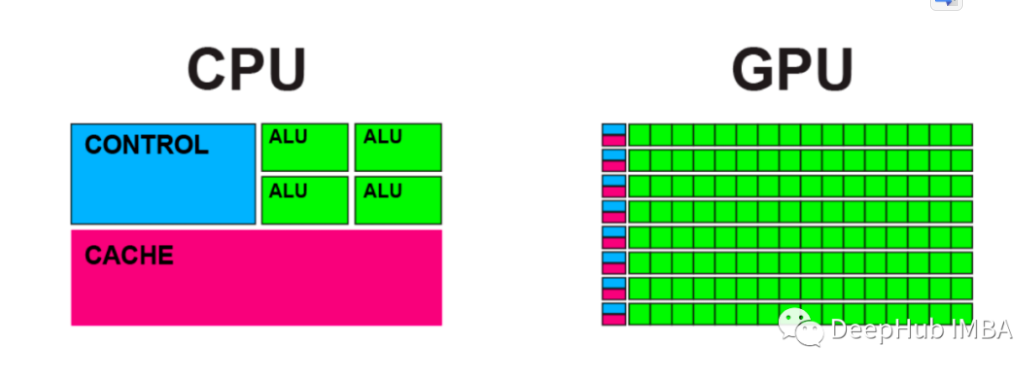CPU：英特尔 i7 6700k (4c/8t) GPU：RTX 3070 TI（6,144 个 CUDA 核心和 192 个 Tensor 核心） 内存：32G 操作系统：Windows 10

#### NVIDIA GPU 术语解释

CUDA 是Compute Unified Device Architecture的缩写。可以使用 CUDA 直接访问 NVIDIA GPU 指令集，与专门为构建游戏引擎而设计的 DirectX 和 OpenGL 不同，CUDA 不需要用户理解复杂的图形编程语言。但是需要说明的是CUDA为N卡独有，所以这就是为什么A卡对于深度学习不友好的原因之一。

Tensor Cores是加速矩阵乘法过程的处理单元。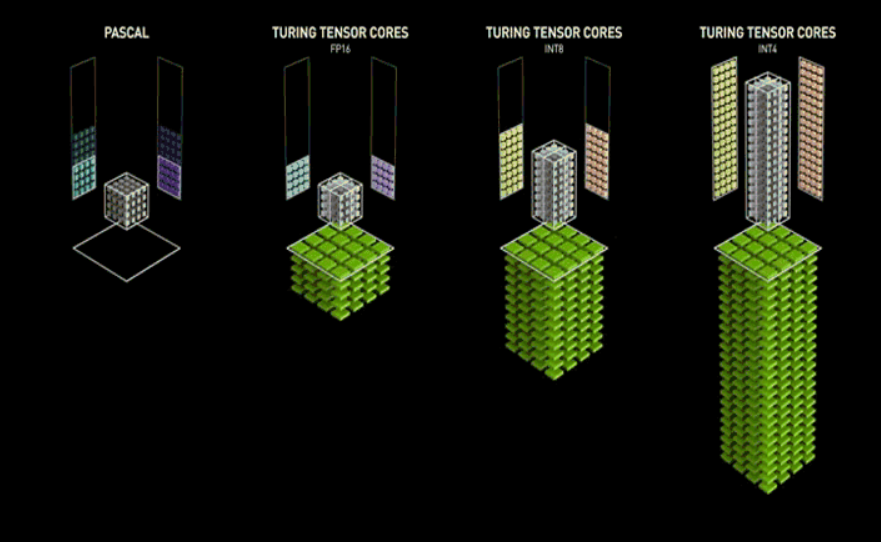CUDA 核心和 Tensor 核心之间有什么关系？Tensor Cores 内置在 CUDA 核心中，当满足某些条件时，就会触发这些核心的操作。

#### 测试方法

GPU的计算速度仅在某些典型场景下比CPU快。在其他的一般情况下，GPU的计算速度可能比CPU慢!但是CUDA在机器学习和深度学习中被广泛使用，因为它在并行矩阵乘法和加法方面特别出色。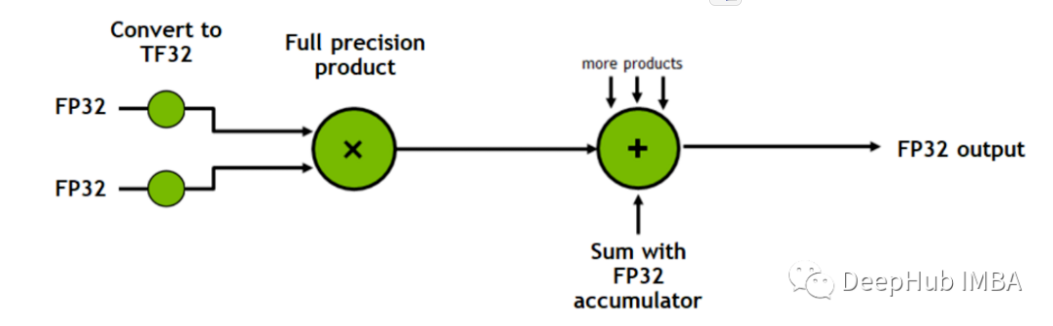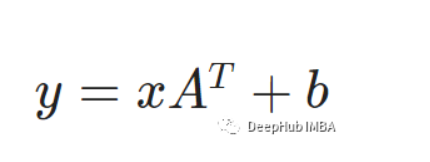````import torch in_row,in_f,out_f = 2,2,3 tensor = torch.randn(in_row,in_f) l_trans = torch.nn.Linear(in_f,out_f) print(l_trans(tensor))`

1.

2.

3.

4.

5.

```

#### CPU 基线测试

````import torch import torch.nn import timein_row, in_f, out_f = 256, 1024, 2048 loop_times = 10000`

1.

2.

3.

4.

```

````s = time.time() tensor = torch.randn(in_row, in_f).to('cpu') l_trans = torch.nn.Linear(in_f, out_f).to('cpu') for _ in range(loop_times): print('cpu take time:',time.time()-s) #cpu take time: 55.70971965789795`

1.

2.

3.

4.

5.

6.

7.

8.

```

#### GPU计算

````s = time.time() tensor = torch.randn(in_row, in_f).cuda() l_trans = torch.nn.Linear(in_f, out_f).cuda() for _ in range(loop_times): torch.cuda.synchronize() print('CUDA take time:',time.time()-s) #CUDA take time: 1.327127456665039`

1.

2.

3.

4.

5.

6.

7.

8.

9.

10.

```

#### 如何使用Tensor Cores

CUDA已经很快了，那么如何启用RTX 3070Ti的197Tensor Cores?，启用后是否会更快呢？在PyTorch中我们需要做的是减少浮点精度从FP32到FP16。，也就是我们说的半精度或者叫混合精度

````s = time.time() tensor = torch.randn(in_row, in_f).cuda().half() layer = torch.nn.Linear(in_f, out_f).cuda().half() for _ in range(loop_times): torch.cuda.synchronize() print('CUDA with tensor cores take time:',time.time()-s) #CUDA with tensor cores take time:0.5381264686584473`

1.

2.

3.

4.

5.

6.

7.

8.

9.

```

#### 总结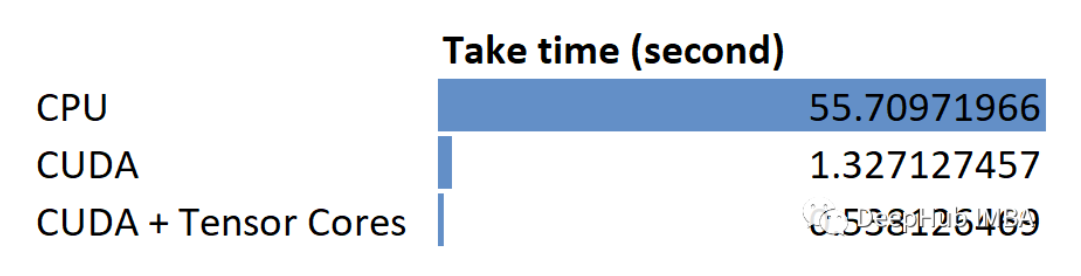NVIDIA的CUDA和Tensor Cores确实大大提高了矩阵乘法的性能。

1、介绍一些简单的CUDA操作（通过Numba），这样可以让我们了解一些细节

2、我们会在拿到4090后发布一个专门针对深度学习的评测，这样可以方便大家购买可选择

12年经验 · 提供上云保障